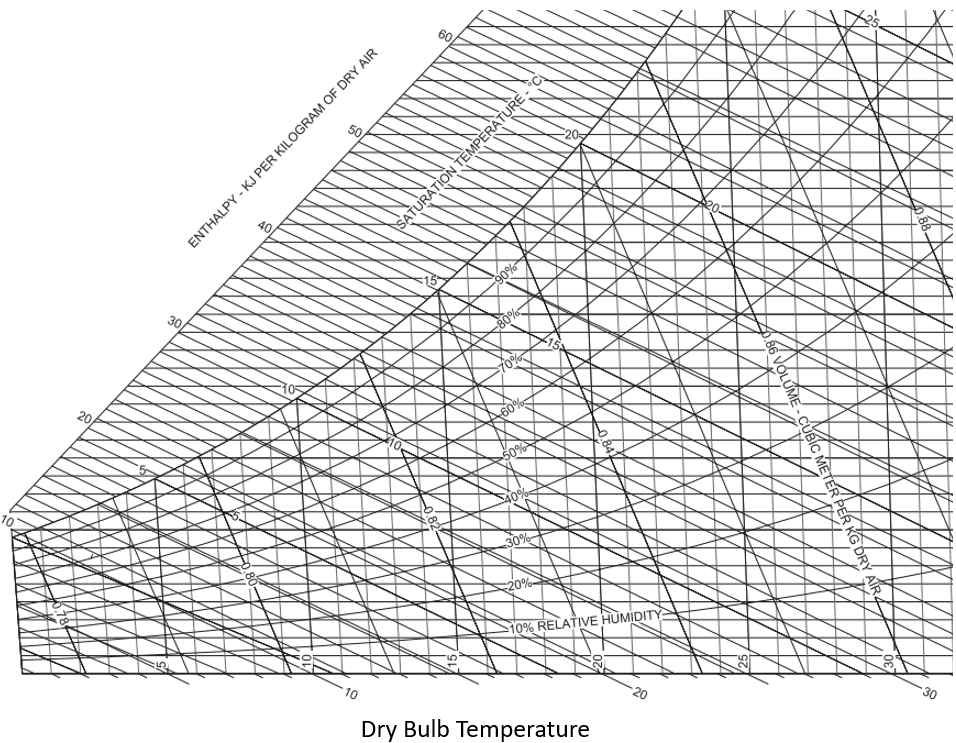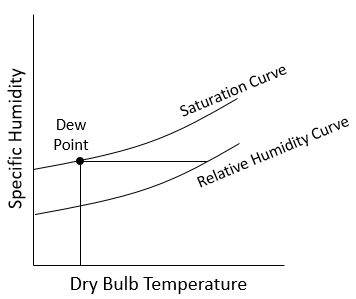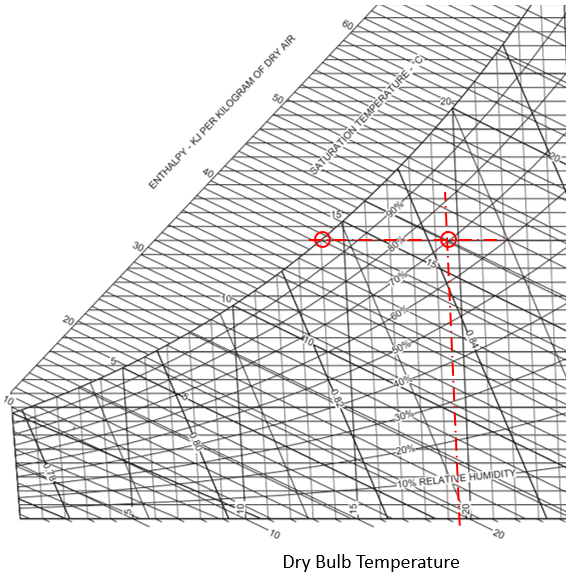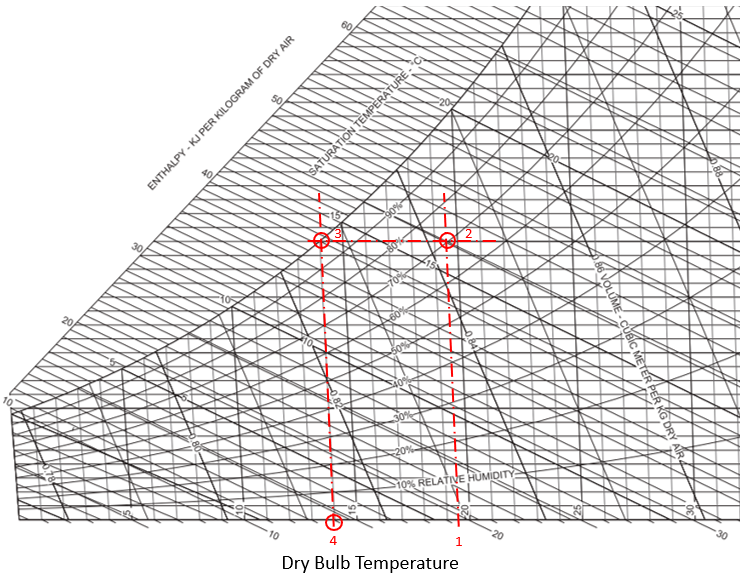## Dew Point

An air conditioning unit pumps dry air into a room at 19.5°C and 70% relative humidity. What is the dew-point temperature?Hint
Properties of an air-water vapor mixture at a fixed pressure are given in graphical form on a psychrometric chart as provided in problem statement. When the system pressure is 1 atm, an ideal-gas mixture is assumed.Hint 2Properties of an air-water vapor mixture at a fixed pressure are given in graphical form on a psychrometric chart as provided in problem statement. When the system pressure is 1 atm, an ideal-gas mixture is assumed.In the psychrometric chart, let’s find where our 19.5°C dry bulb temperature intersects with the 70% relative humidity curve (2). From there, follow the line horizontally left until the saturation curve is reached (3). This is the dew point. Read down to find the dew-point temperature (4).The dew point temperature is 14°C.
14°C